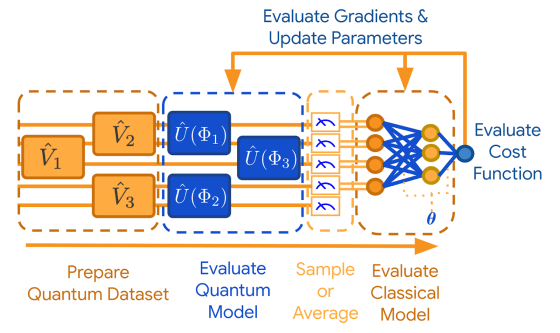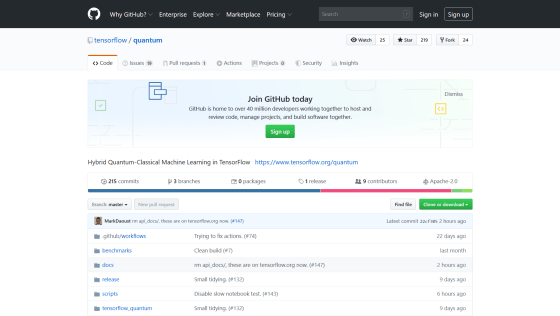# Google releases machine learning library `` TensorFlow Quantum '' corresponding to quantum dataGoogle has released a library for quantum machine learning, ' TensorFlow Quantum (TFQ) ', in collaboration with

Waterloo University , Volkswagen, and a secret research institute ' X '. TFQ is a tool that connects the quantum computing and machine learning research communities and models the structure of quantum.

[2003.02989] TensorFlow Quantum: A Software Framework for Quantum Machine Learning
https://arxiv.org/abs/2003.02989

Google AI Blog: Announcing TensorFlow Quantum: An Open Source Library for Quantum Machine Learning

TensorFlow Quantum
https://www.tensorflow.org/quantum

TFQ integrates the framework ' Cirq ' developed by Google for the quantum processor ' NISQ ' with quantum noise into the existing TensorFlow library. Quantum computing compatible with the existing TensorFlow API is realized.

In order to understand the model of quantum machine learning, it is necessary to first understand 'quantum data' and 'quantum classic hybrid model'. 'Quantum computer' and 'quantum data' are explained in detail in the following articles.

How do experts explain 'quantum computers' to people with different knowledge and experience? -GIGAZINEQuantum data is data generated by NISQ that has '

quantum entanglement ' in which quanta overlap or entangle. Quantum data contains noise, but if quantum machine learning is applied to noisy quantum data, classical information can be extracted to the maximum. TFQ is a library focusing on this property, and provides the basis for extracting and generalizing quantum data.

Since NISQ is noisy and small in scale, it can be used in conjunction with classical processors to perform efficient calculations, but the quantum classical hybrid model enables such calculations. TFQ the qubit and the quantum gate , a quantum circuit by mounting a compiler or scheduler for NISQ contained in addition to the common structure are used in such hitherto quantum computing, Cirq, efficient It is said that a quantum classical hybrid model has been realized.

When TFQ processes quantum data, it first evaluates the data using a quantum model. Here, we extract quantum data with entanglement and extract information in a classical form hidden in the overlap. Random variables in the classical form representing quantum states are sampled or averaged, and neural networks process the data using classical computing. Then each variable is updated to optimize the objective function .The feature of TFQ is that a large number of quantum circuits can be calculated in parallel, and a relatively large quantum circuit can be simulated by a multi-core processor. In particular, to realize the latter, it has adopted

`` qsim '', a high-performance quantum circuit simulator optimized for Intel's multi-core processor, so that the GCP N2 node reduces the vCPU to 80 cores When set, it is reported that 1 million simulations could be performed with 20 quantum circuits with a depth of 20 in 60 minutes.

At the release stage, TFQ uses the existing quantum circuit simulator, but it is commented that it will be compatible with actual quantum processors such as the quantum processor ' Sycamore ' developed by Google in the future.

TFQ is published on GitHub as open source software.

GitHub-tensorflow / quantum: Hybrid Quantum-Classical Machine Learning in TensorFlow
https://github.com/tensorflow/quantumin Software, Posted by darkhorse_log